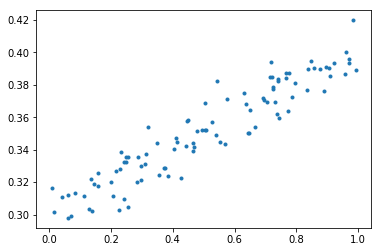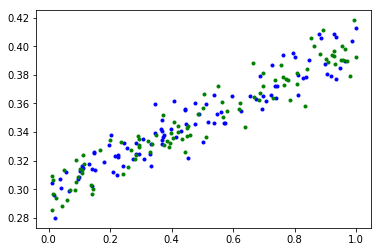!pip3 install tensorflow

Collecting tensorflow
100% |████████████████████████████████| 31.0MB 12kB/s  eta 0:00:01   19% |██████▎                         | 6.1MB 39.2MB/s eta 0:00:01    57% |██████████████████▌             | 17.9MB 40.1MB/s eta 0:00:01
Requirement already satisfied: numpy>=1.11.0 in /srv/paws/lib/python3.4/site-packages (from tensorflow)
Requirement already satisfied: werkzeug>=0.11.10 in /srv/paws/lib/python3.4/site-packages (from tensorflow)
Collecting wheel>=0.26 (from tensorflow)
100% |████████████████████████████████| 71kB 1.9MB/s eta 0:00:01
Collecting protobuf>=3.2.0 (from tensorflow)
100% |████████████████████████████████| 5.7MB 71kB/s  eta 0:00:01
Requirement already satisfied: six>=1.10.0 in /srv/paws/lib/python3.4/site-packages (from tensorflow)
Requirement already satisfied: setuptools in /srv/paws/lib/python3.4/site-packages (from protobuf>=3.2.0->tensorflow)
Requirement already satisfied: packaging>=16.8 in /srv/paws/lib/python3.4/site-packages (from setuptools->protobuf>=3.2.0->tensorflow)
Requirement already satisfied: appdirs>=1.4.0 in /srv/paws/lib/python3.4/site-packages (from setuptools->protobuf>=3.2.0->tensorflow)
Requirement already satisfied: pyparsing in /srv/paws/lib/python3.4/site-packages (from packaging>=16.8->setuptools->protobuf>=3.2.0->tensorflow)
Installing collected packages: wheel, protobuf, tensorflow
Successfully installed protobuf-3.3.0 tensorflow-1.1.0 wheel-0.29.0

import tensorflow as tf
print("You have version %s" % tf.__version__)

You have version 1.1.0

# The next three imports help with compatability between
# Python 2 and 3
from __future__ import absolute_import
from __future__ import division
from __future__ import print_function

import os

import numpy as np
import pylab
import tensorflow as tf

# A special command for IPython Notebooks that
# intructs Matplotlib to display plots in the notebook
%matplotlib inline

# This is a directory we'll use to store information
# about the graph to later visualize in TensorBoard.
# By default, it will be created in the same directory
# as this notebook.

# Be sure to delete the contents of this directory before
# running the script.
LOGDIR = './graphs'

%matplotlib inline
import pylab
import numpy as np

# create some data using numpy. y = x * 0.1 + 0.3 + noise
x = np.random.rand(100).astype(np.float32)
noise = np.random.normal(scale=0.01, size=len(x))
y = x * 0.1 + 0.3 + noise

# plot it
pylab.plot(x, y, '.')

[<matplotlib.lines.Line2D at 0x7faee421f048>]tf.reset_default_graph()
sess = tf.Session()

def make_noisy_data(m=0.1, b=0.3, n=100):
x = np.random.rand(n).astype(np.float32)
noise = np.random.normal(scale=0.01, size=len(x))
y = m * x + b + noise
return x, y

x_train, y_train = make_noisy_data()
x_test, y_test = make_noisy_data()

# Uncomment the following lines to plot the data.
pylab.plot(x_train, y_train, 'b.')
pylab.plot(x_test, y_test, 'g.')

[<matplotlib.lines.Line2D at 0x7faee42630b8>]# tf.name_scope is used to make a graph legible in the TensorBoard graph explorer
# shape=[None] means x_placeholder is a one dimensional array of any length.
# name='x' gives TensorBoard a display name for this node.
with tf.name_scope('input'):
x_placeholder = tf.placeholder(shape=[None], dtype=tf.float32, name='x-input')
y_placeholder = tf.placeholder(shape=[None], dtype=tf.float32, name='y-input')

with tf.name_scope('model'):
m = tf.Variable(tf.random_normal(), name='m')
b = tf.Variable(tf.random_normal(), name='b')
# This is the same as y = tf.add(tf.mul(m, x_placeholder), b), but looks nicer
y = m * x_placeholder + b

# Uncomment the following lines to see what m, b, and y are
print(m)
print(b)
print(y)

<tf.Variable 'model/m:0' shape=(1,) dtype=float32_ref>
<tf.Variable 'model/b:0' shape=(1,) dtype=float32_ref>

LEARNING_RATE = 0.5

with tf.name_scope('training'):
with tf.name_scope('loss'):
loss = tf.reduce_mean(tf.square(y - y_placeholder))
with tf.name_scope('optimizer'):
train = optimizer.minimize(loss)

# Uncomment the following 3 lines to see what 'loss', 'optimizer' and 'train' are.
print("loss:", loss)
print("optimizer:", optimizer)
print("train_step:", train)

loss: Tensor("training_1/loss/Mean:0", shape=(), dtype=float32)
op: "NoOp"


# Write the graph
writer = tf.summary.FileWriter(LOGDIR)

sess.run(tf.global_variables_initializer())